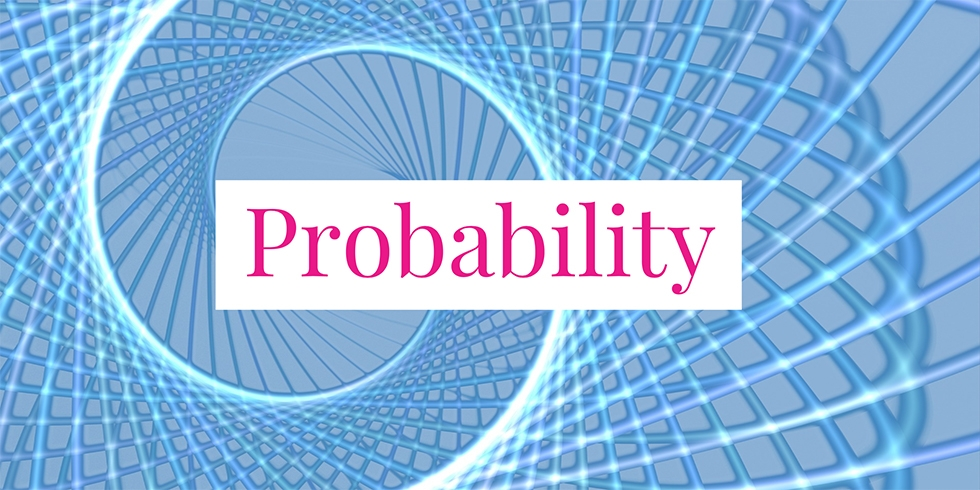# Conditional Probability When the Sum of Two Geometric Random Variables Are Known## Problem 755

Let $X$ and $Y$ be geometric random variables with parameter $p$, with $0 \leq p \leq 1$. Assume that $X$ and $Y$ are independent.

Let $n$ be an integer greater than $1$. Let $k$ be a natural number with $k\leq n$. Then prove the formula
$P(X=k \mid X + Y = n) = \frac{1}{n-1}.$Add to solve later

## Hint.

If this problem is a bit abstract for you, then you might want to try the following problem, which is more concrete.

Probability that Alice Tossed a Coin Three Times If Alice and Bob Tossed Totally 7 Times

## Solution.

The definition of a conditional probability yields
$P(X=k \mid X + Y = n) = \frac{P\left((X=k) \cap (X+Y=n)\right)}{P(X+Y=n)} \tag{*}$ We first compute the numerator of the last expression. We have
\begin{align*}
&P\left((X=k) \cap (X+Y=n)\right)\\
&= P\left((X=k) \cap (Y=n-X)\right)\\
&= P\left((X=k) \cap (Y=n-k)\right)\\
&= P(X=k) \cdot P(Y=n-k).
\end{align*}
Here, the last step follows because $X$ and $Y$ are independent.

Now, as $X$ and $Y$ are geometric random variables with parameter $p$, we see that by putting $q=1-p$
\begin{align*}
P(X=k) &= q^{k-1}p \\
P(Y= n – k) &= q^{n-k-1}p.
\end{align*}
Hence, the numerator becomes
\begin{align*}
&P\left((X=k) \cap (X+Y=n)\right)\\
&= P(X=k) \cdot P(Y=n-k)\\
&= q^{k-1}p \cdot q^{n-k-1}p\\
&= q^{n-2}p^2.
\end{align*}

Next, we compute the denominator of (*). Applying the law of total probability, we get
\begin{align*}
P(X+Y=n) &= \sum_{k=1}^{n-1} P(X=k) P(X+Y=n \mid X=k). \tag{**}
\end{align*}
The probability $P(X+Y=n \mid X=k)$ can be computed as follows.
\begin{align*}
&P(X+Y=n \mid X=k)\\
&= P(Y = n-X \mid X=k)\\
&= P(Y=n-k \mid X=k)\\
&= P(Y=n-k).
\end{align*}
The last step follows because $X$ and $Y$ are independent. Then the equation (**) becomes
\begin{align*}
P(X+Y=n) &= \sum_{k=1}^{n-1} P(X=k) \cdot P(Y=n-k) \\ &= \sum_{k=1}^{n-1} q^{k-1}p \cdot q^{n-k-1}p\\
&= \sum_{k=1}^{n-1} q^{n-2} p^2\\
&= (n-1)q^{n-2}p^2.
\end{align*}

Combining the computations of the numerator and the denominator, we obtain the desired probability from (*).
\begin{align*}
P(X=k \mid X + Y = n) &= \frac{P\left((X=k) \cap (X+Y=n)\right)}{P(X+Y=n)}\\
&= \frac{q^{n-2}p^2}{(n-1)q^{n-2}p^2}\\
&= \frac{1}{n-1}.
\end{align*}
This completes the proof of the formula
$P(X=k \mid X + Y = n) = \frac{1}{n-1}.$

## Application

As an application of this problem, solve the next problem.

Problem.
Alice tossed a fair coin until a head occurred. Then Bob tossed the coin until a head occurred. Suppose that the total number of tosses for Alice and Bob was $7$.

Assuming that each toss is independent of each other, what is the probability that Alice tossed the coin exactly three times?

Its solution is available in the post Probability that Alice Tossed a Coin Three Times If Alice and Bob Tossed Totally 7 Times.Add to solve later

### 1 Response

1. 02/05/2020

[…] Its solution can be found in the post: Conditional Probability When the Sum of Two Geometric Random Variables Are Known […]

This site uses Akismet to reduce spam. Learn how your comment data is processed.

###### More in Probability##### Probability that Three Pieces Form a Triangle

We have a stick of a unit length. Two points on the stick will be selected randomly (uniformly along the...

Close